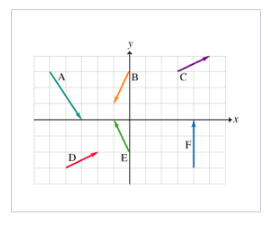# Problem: Adding and Subtracting Vectors Conceptual QuestionSix vectors ( A to F) have the magnitudes and directions indicated in the figure. Which two vectors, when added, will have the largest (positive) x component?1. C &amp; E2. E &amp; F3. A &amp; F4. C &amp; D5. B &amp; D

###### FREE Expert Solution

A = 2i - 3j

B = -1i - 2j

C = 2i + 1j

D = 2i + 1j

E = -1i + 2j

F = 0 i + 3j

Sum of x-componets:

1. 2i - 1i = 1i

2. -1i + 0i = -1i###### Problem Details

Adding and Subtracting Vectors Conceptual Question

Six vectors ( A to F) have the magnitudes and directions indicated in the figure.Which two vectors, when added, will have the largest (positive) x component?

1. C & E

2. E & F

3. A & F

4. C & D

5. B & D